# Sketch and Constrain Bracket Graphic Inputs - Intergraph Smart 3D - Reference Data

## Intergraph Smart 3D 2D Symbols

PPMProductFamily
3D Design and Visualization
PPMProduct
Intergraph Smart 3D
PPMCategory
Reference Data
Version_S3D
12.1(2019)
Version_SPEM_custom
9.0.0
Version_SSK
10.0(2018)

Bracket symbols contain between two and five geometric inputs. To make the symbol as flexible as possible, the graphic inputs should be defined as B-spline curves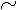. The first two of these graphic inputs will serve as the U- and V-axes of the symbol. The graphic inputs must be defined according to the following rules:

• B-spline curves should be defined using three or four points.

• The first two B-splines curves forming the U- and V-axes should be much longer than the bracket arm geometry.

• The first two B-spline curves do not need to share a common endpoint.

• The first two B-spline curves should not be constrained together.

• The symbol geometry must be drawn in the (+U,+V) quadrant of the coordinate axes defined by the first two curves.

• All B-spline curves serving as graphic inputs must be locked at their midpoints.

Inputs go on one of the following layers:

• Inputs - Used for supports that do not bound the bracket.

• Trim_By - Used for supports that bound (that is, trim) the bracket.

1. Make either the Inputs or Trim_By layer active.

2. Locate the (0,0) point in the 2D Symbols file by placing a point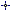at (0,0).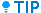The point is easier to find for later reference if you place a small circle centered on the point.

3. Create the first curve approximately parallel to the +X axis, beginning just to the left of the (0,0) point and passing as close as possible to it.

4. Create the next curve approximately parallel to the +Y axis, beginning just below the (0,0) point and passing as close as possible to it.

5. Lock the curves with the Lock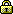command by clicking near each curve's midpoint.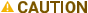Do not lock the ends of the curves.

6. Delete the (0,0) point and circle created in Step 2.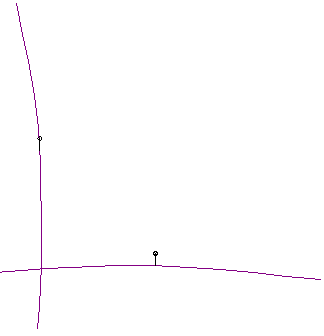7. Create all other required support and limit graphical inputs in their approximate positions relative to the U-and V-axis inputs in a manner similar to that previously described in this section.

8. If some of the support curves belong on the other input layer, shift-click to select the curves.

9. Click Change Layer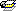on the Layer toolbar.

10. On the dialog box, select the needed layer (Inputs or Trim_By) and click OK.